# Approximate n-Jordan ∗-derivations on ${C}^{\ast }$-algebras and $J{C}^{\ast }$-algebras

## Abstract

In this paper, we investigate the superstability and the Hyers-Ulam stability of n-Jordan -derivations on ${C}^{\ast }$-algebras and $J{C}^{\ast }$-algebras.

MSC:17C65, 39B52, 39B72, 46L05.

## 1 Introduction and preliminaries

The stability of functional equations was first introduced by Ulam  in 1940. More precisely, he proposed the following problem. Given a group ${H}_{1}$, a metric group $\left({H}_{2},d\right)$ and $ϵ>0$, does there exist a $\delta >0$ such that if a mapping $f:{H}_{1}\to {H}_{2}$ satisfies the inequality $d\left(f\left(xy\right),f\left(x\right)f\left(y\right)\right)<\delta$ for all $x,y\in {H}_{1}$, then there exists a homomorphism $T:{H}_{1}\to {H}_{2}$ such that $d\left(f\left(x\right),T\left(x\right)\right)<ϵ$ for all $x\in {H}_{1}$? As mentioned above, when this problem has a solution, we say that the homomorphisms from ${H}_{1}$ to ${H}_{2}$ are stable. In 1941, Hyers  gave a partial solution of the Ulam problem for the case of approximate additive mappings under the assumption that ${H}_{1}$ and ${H}_{2}$ are Banach spaces. In 1950, Aoki  generalized the Hyers theorem for approximately additive mappings. In 1978, Rassias  generalized the theorem of Hyers by considering the stability problem with unbounded Cauchy differences. During the last decades, several stability problems of functional equations have been investigated by many mathematicians (see ).

Let n be an integer greater than one and A be a ring, and let B be an A-module. An additive mapping $D:A\to B$ is called an n-Jordan derivation (resp. n-ring derivation) if

$D\left({a}^{n}\right)=D\left(a\right){a}^{n-1}+aD\left(a\right){a}^{n-2}+\cdots +{a}^{n-2}D\left(a\right)a+{a}^{n-1}D\left(a\right)$

for all $a\in A$

$\left(\text{resp.}\phantom{\rule{1em}{0ex}}D\left(\prod _{i=1}^{n}{a}_{i}\right)=D\left({a}_{1}\right){a}_{2}\cdots {a}_{n}+{a}_{1}D\left({a}_{2}\right){a}_{3}\cdots {a}_{n}+\cdots +{a}_{1}{a}_{2}\cdots {a}_{n-1}D\left({a}_{n}\right)$

for all ${a}_{1},{a}_{2},\dots ,{a}_{n}\in A$). The concept of n-Jordan derivations was studied by Eshaghi Gordji  (see also ).

Definition 1.1 Let A be a ${C}^{\ast }$-algebra. A -linear mapping $D:A\to A$ is called an n-Jordan -derivation if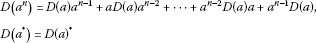for all $a\in A$.

A ${C}^{\ast }$-algebra A, endowed with the Jordan product $a\circ b=\frac{ab+ba}{2}$ on A, is called a $J{C}^{\ast }$-algebra (see ).

Definition 1.2 Let A be a $J{C}^{\ast }$-algebra. A -linear mapping $D:A\to A$ is called an n-Jordan -derivation if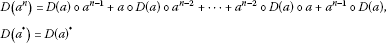for all $a\in A$.

This paper is organized as follows. In Section 2, we investigate the superstability of n-Jordan -derivations on ${C}^{\ast }$-algebras associated with the following functional inequality:

$\parallel f\left(x\right)+f\left(y\right)+f\left(z\right)\parallel \le \parallel 2f\left(\frac{x+y+z}{2}\right)\parallel .$
(1.1)

We, moreover, prove the Hyers-Ulam stability of n-Jordan -derivations on ${C}^{\ast }$-algebras associated with the following functional equation:

$2f\left(\frac{x+y}{2}\right)=f\left(x\right)+f\left(y\right).$
(1.2)

In Section 3, we investigate the superstability of n-Jordan -derivations on $J{C}^{\ast }$-algebras associated with the functional inequality (1.1) and prove the Hyers-Ulam stability of n-Jordan -derivations on $J{C}^{\ast }$-algebras associated with the functional equation (1.2).

In this paper, assume that n is an integer greater than one.

## 2 n-Jordan ∗-derivations on ${C}^{\ast }$-algebras

Throughout this section, assume that A is a ${C}^{\ast }$-algebra, and that ${n}_{0}$ is a fixed positive integer.

Lemma 2.1 Let $f:A\to A$ be a mapping such that

$\parallel \mu f\left(x\right)+\mu f\left(y\right)+f\left(\mu z\right)\parallel \le \parallel 2f\left(\mu \frac{x+y+z}{2}\right)\parallel$
(2.1)

for all $\mu \in {\mathbb{T}}_{{n}_{0}}^{1}:=\left\{{e}^{i\theta }\in \mathbb{C}:0\le \theta \le \frac{2\pi }{{n}_{0}}\right\}$ and all $x,y,z\in A$. Then the mapping $f:A\to A$ is -linear.

Proof Letting $\mu =1$ and $x=y=z=0$ in (2.1), we get

$\parallel 3f\left(0\right)\parallel \le 2\parallel f\left(0\right)\parallel .$

So, $f\left(0\right)=0$.

Letting $\mu =1$, $y=-x$ and $z=0$ in (2.1), we get

$\parallel f\left(x\right)+f\left(-x\right)\parallel \le \parallel 2f\left(0\right)\parallel =0$

and so $f\left(-x\right)=-f\left(x\right)$ for all $x\in A$.

Letting $\mu =1$ and $z=-x-y$ in (2.1), we get

$\parallel f\left(x\right)+f\left(y\right)+f\left(-x-y\right)\parallel \le \parallel 2f\left(0\right)\parallel =0$

and so

$f\left(x+y\right)=-f\left(-x-y\right)=f\left(x\right)+f\left(y\right)$

for all $x,y\in A$. Hence, the mapping $f:A\to A$ is additive.

Letting $y=0$ and $z=-x$ in (2.1), we get

$\parallel \mu f\left(x\right)+f\left(-\mu x\right)\parallel \le \parallel 2f\left(0\right)\parallel =0$

for all $x\in A$ and all $\mu \in {\mathbb{T}}_{{n}_{0}}^{1}$. So, $f\left(\mu x\right)=\mu f\left(x\right)$ for all $x\in A$ and all $\mu \in {\mathbb{T}}_{{n}_{0}}^{1}$. By [, Lemma 2.1], the mapping $f:A\to A$ is -linear. □

We prove the superstability of n-Jordan -derivations on ${C}^{\ast }$-algebras.

Theorem 2.2 Let $f:A\to A$ be a mapping satisfying (2.1) and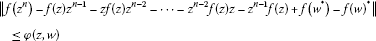(2.2)

for all $z,w\in A$, where $\phi :A×A\to \left[0,\mathrm{\infty }\right)$ satisfies

$\sum _{i=0}^{\mathrm{\infty }}\frac{1}{{2}^{i}}\phi \left({2}^{i}z,{2}^{i}w\right)<\mathrm{\infty }\phantom{\rule{1em}{0ex}}\mathit{\text{or}}\phantom{\rule{1em}{0ex}}\sum _{i=0}^{\mathrm{\infty }}{2}^{i}\phi \left(\frac{z}{{2}^{i}},\frac{w}{{2}^{i}}\right)<\mathrm{\infty }$
(2.3)

for all $z,w\in A$. Then the mapping $f:A\to A$ is an n-Jordan -derivation.

Proof By Lemma 2.1, the mapping $f:A\to A$ is -linear.

Assume that $\phi :A×A\to \left[0,\mathrm{\infty }\right)$ satisfies ${\sum }_{i=0}^{\mathrm{\infty }}\frac{1}{{2}^{i}}\phi \left({2}^{i}z,{2}^{i}w\right)<\mathrm{\infty }$ for all $z,w\in A$.

It follows from (2.2) that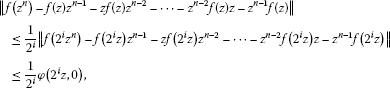which tends to zero as $i\to \mathrm{\infty }$ for all $z\in A$. So,

$f\left({z}^{n}\right)=f\left(z\right){z}^{n-1}+zf\left(z\right){z}^{n-2}+\cdots +{z}^{n-2}f\left(z\right)z+{z}^{n-1}f\left(z\right)$

for all $z\in A$.

Similarly, one can show that

$f\left({w}^{\ast }\right)=f{\left(w\right)}^{\ast }$

for all $w\in A$.

Therefore, the mapping $f:A\to A$ is an n-Jordan -derivation.

Assume that $\phi :A×A\to \left[0,\mathrm{\infty }\right)$ satisfies ${\sum }_{i=0}^{\mathrm{\infty }}{2}^{i}\phi \left(\frac{z}{{2}^{i}},\frac{w}{{2}^{i}}\right)<\mathrm{\infty }$ for all $z,w\in A$. By the same reasoning as in the previous case, one can prove that the mapping $f:A\to A$ is an n-Jordan -derivation. □

Corollary 2.3 Let $p\ne 1$ and θ be nonnegative real numbers. Let $f:A\to A$ be a mapping satisfying (2.1) and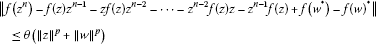for all $z,w\in A$. Then the mapping $f:A\to A$ is an n-Jordan -derivation.

Now we prove the Hyers-Ulam stability of n-Jordan derivations on ${C}^{\ast }$-algebras.

Theorem 2.4 Let $f:A\to A$ be a mapping with $f\left(0\right)=0$ for which there exists a function $\phi :{A}^{4}\to \left[0,\mathrm{\infty }\right)$ such that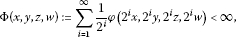(2.4)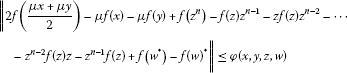(2.5)

for all $x,y,z,w\in A$ and all $\mu \in {\mathbb{T}}_{{n}_{0}}^{1}$. Then there exists a unique n-Jordan -derivation $D:A\to A$ such that

$\parallel f\left(x\right)-D\left(x\right)\parallel \le \mathrm{\Phi }\left(x,0,0,0\right)$
(2.6)

for all $x\in A$.

Proof Letting $\mu =1$, $y=z=w=0$ and replacing x by 2x in (2.5), we get

$\parallel f\left(x\right)-\frac{1}{2}f\left(2x\right)\parallel \le \frac{1}{2}\phi \left(2x,0,0,0\right)$
(2.7)

for all $x\in A$.

Using the induction method, we have

$\parallel f\left(x\right)-\frac{1}{{2}^{l}}f\left({2}^{l}x\right)\parallel \le \sum _{i=1}^{l}\frac{1}{{2}^{i}}\phi \left({2}^{i}x,0,0,0\right)$
(2.8)

for all $x\in A$. Replacing x by ${2}^{m}x$ in (2.8) and multiplying by $\frac{1}{{2}^{m}}$, we have

$\parallel \frac{1}{{2}^{m}}f\left({2}^{m}x\right)-\frac{1}{{2}^{l+m}}f\left({2}^{l+m}x\right)\parallel \le \sum _{i=m+1}^{l+m}\frac{1}{{2}^{i}}\phi \left({2}^{i}x,0,0,0\right)$

for all $x\in A$. Hence, $\left\{\frac{1}{{2}^{l}}f\left({2}^{l}x\right)\right\}$ is a Cauchy sequence. Since A is complete,

$D\left(x\right)=\underset{l\to \mathrm{\infty }}{lim}\frac{1}{{2}^{l}}f\left({2}^{l}x\right)$

exists for all $x\in A$.

Taking the limit as $l\to \mathrm{\infty }$ in (2.8), we obtain the inequality (2.6).

It follows from (2.4) and (2.5) that

$\begin{array}{rcl}\parallel 2D\left(\frac{x+y}{2}\right)-D\left(x\right)-D\left(y\right)\parallel & =& \underset{l\to \mathrm{\infty }}{lim}\frac{1}{{2}^{l}}\parallel 2f\left({2}^{l-1}\left(x+y\right)\right)-f\left({2}^{l}x\right)-f\left({2}^{l}y\right)\parallel \\ \le & \underset{l\to \mathrm{\infty }}{lim}\frac{1}{{2}^{l}}\phi \left({2}^{l}x,{2}^{l}y,0,0\right)\end{array}$

for all $x,y\in A$. So,

$2D\left(\frac{x+y}{2}\right)=D\left(x\right)+D\left(y\right)$

for all $x,y\in A$. Since $f\left(0\right)=0$, $D\left(0\right)=0$. Thus, D is additive.

To prove the uniqueness, let L be another additive mapping satisfying (2.6). Then we have, for any positive integer k,

$\begin{array}{rcl}\parallel D\left(x\right)-L\left(x\right)\parallel & \le & \frac{1}{{2}^{k}}\left(\parallel D\left({2}^{k}x\right)-f\left({2}^{k}x\right)\parallel +\parallel f\left({2}^{k}x\right)-L\left({2}^{k}x\right)\parallel \right)\\ \le & \frac{2}{{2}^{k}}\mathrm{\Phi }\left({2}^{k}x,0,0,0\right),\end{array}$

which tends to zero as $k\to \mathrm{\infty }$. So, we conclude that $D\left(x\right)=L\left(x\right)$ for all $x\in A$.

On the other hand, we have

$D\left(\mu x\right)-\mu D\left(x\right)=\underset{\mathrm{ł}\to \mathrm{\infty }}{lim}\frac{1}{{2}^{l}}\parallel f\left({2}^{l}\mu x\right)-\mu f\left({2}^{l}x\right)\parallel \le \underset{l\to \mathrm{\infty }}{lim}\frac{1}{{2}^{l+1}}\phi \left({2}^{l}x,{2}^{l}x,0,0\right)=0$

for all $\mu \in {\mathbb{T}}_{{n}_{0}}^{1}$ and all $x\in A$. By [, Lemma 2.1], the mapping $D:A\to A$ is -linear.

It follows from (2.4) and (2.5) thatand

$\parallel D\left({w}^{\ast }\right)-D{\left(w\right)}^{\ast }\parallel =\underset{m\to \mathrm{\infty }}{lim}\parallel \frac{1}{{2}^{m}}f\left({2}^{m}{w}^{\ast }\right)-\frac{1}{{2}^{m}}{\left(f\left({2}^{m}w\right)\right)}^{\ast }\parallel \le \underset{m\to \mathrm{\infty }}{lim}\frac{1}{{2}^{m}}\phi \left(0,0,0,{2}^{n}w\right)=0$

for all $z,w\in A$. Hence, $D:A\to A$ is a unique n-Jordan -derivation. □

Corollary 2.5 Let $f:A\to A$ be a mapping with $f\left(0\right)=0$ for which there exist positive constants θ and $p<1$ such that(2.9)

for all $x,y,z,w\in A$ and all $\mu \in {\mathbb{T}}_{{n}_{0}}^{1}$. Then there exists a unique n-Jordan -derivation $D:A\to A$ such that

$\parallel f\left(x\right)-D\left(x\right)\parallel \le \frac{{2}^{p}\theta }{2-{2}^{p}}{\parallel x\parallel }^{p}$

for all $x\in A$.

Proof Letting $\phi \left(x,y,z,w\right)=\theta \left({\parallel x\parallel }^{p}+{\parallel y\parallel }^{p}+{\parallel z\parallel }^{p}+{\parallel w\parallel }^{p}\right)$ in Theorem 2.4, we have

$\parallel f\left(x\right)-D\left(x\right)\parallel \le \frac{{2}^{p}\theta }{2-{2}^{p}}{\parallel x\parallel }^{p}$

for all $x\in A$, as desired. □

Theorem 2.6 Let $f:A\to A$ be a mapping for which there exists a function $\phi :{A}^{4}\to \left[0,\mathrm{\infty }\right)$ satisfying (2.5) and

$\sum _{i=0}^{\mathrm{\infty }}{2}^{ni}\phi \left(\frac{x}{{2}^{i}},\frac{y}{{2}^{i}},\frac{z}{{2}^{i}},\frac{w}{{2}^{i}}\right)<\mathrm{\infty }$
(2.10)

for all $x,y,z,w\in A$. Then there exists a unique n-Jordan -derivation $D:A\to A$ such that

$\parallel f\left(x\right)-D\left(x\right)\parallel \le \sum _{i=0}^{\mathrm{\infty }}{2}^{i}\phi \left(\frac{x}{{2}^{i}},0,0,0\right)$
(2.11)

for all $x\in A$.

Proof It follows from (2.7) that

$\parallel f\left(x\right)-2f\left(\frac{x}{2}\right)\parallel \le \phi \left(x,0,0,0\right)$

for all $x\in A$.

The rest of the proof is similar to the proof of Theorem 2.4. □

Corollary 2.7 Let $f:A\to A$ be a mapping with $f\left(0\right)=0$ for which there exist positive constants θ and $p>n$ satisfying (2.9). Then there exists a unique n-Jordan -derivation $D:A\to A$ such that

$\parallel f\left(x\right)-D\left(x\right)\parallel \le \frac{{2}^{p}\theta }{{2}^{p}-2}{\parallel x\parallel }^{p}$

for all $x\in A$.

Proof Letting $\phi \left(x,y,z,w\right)=\theta \left({\parallel x\parallel }^{p}+{\parallel y\parallel }^{p}+{\parallel z\parallel }^{p}+{\parallel w\parallel }^{p}\right)$ in Theorem 2.6, we have

$\parallel f\left(x\right)-D\left(x\right)\parallel \le \frac{{2}^{p}\theta }{{2}^{p}-2}{\parallel x\parallel }^{p}$

for all $x\in A$, as desired. □

## 3 n-Jordan ∗-derivations on $J{C}^{\ast }$-algebras

Throughout this section, assume that A is a $J{C}^{\ast }$-algebra.

We prove the superstability of n-Jordan -derivations on $J{C}^{\ast }$-algebras.

Theorem 3.1 Let $f:A\to A$ be a mapping satisfying (2.1) and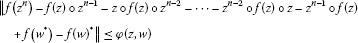for all $z,w\in A$, where $\phi :A×A\to \left[0,\mathrm{\infty }\right)$ satisfies (2.3). Then the mapping $f:A\to A$ is an n-Jordan -derivation.

Proof The proof is similar to the proof of Theorem 2.2. □

Corollary 3.2 Let $p\ne 1$ and θ be nonnegative real numbers. Let $f:A\to A$ be a mapping satisfying (2.1) and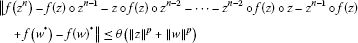for all $z,w\in A$. Then the mapping $f:A\to A$ is an n-Jordan -derivation.

We prove the Hyers-Ulam stability of n-Jordan derivations on $J{C}^{\ast }$-algebras.

Theorem 3.3 Let $f:A\to A$ be a mapping with $f\left(0\right)=0$ for which there exists a function $\phi :{A}^{4}\to \left[0,\mathrm{\infty }\right)$ satisfying (2.4) and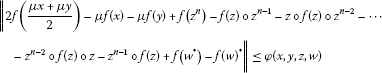(3.1)

for all $x,y,z,w\in A$ and all $\mu \in {\mathbb{T}}_{{n}_{0}}^{1}$. Then there exists a unique n-Jordan -derivation $D:A\to A$ satisfying (2.6).

Proof By the same reasoning as in the proof of Theorem 2.4, there exists a unique -linear $D:A\to A$ such that

$\parallel f\left(x\right)-D\left(x\right)\parallel \le \mathrm{\Phi }\left(x,0,0,0\right)$

for all $x\in A$. The mapping $D:A\to A$ is given by

$D\left(x\right)=\underset{l\to \mathrm{\infty }}{lim}\frac{1}{{2}^{l}}f\left({2}^{l}x\right)$

for all $x\in A$.

The rest of the proof is similar to the proof of Theorem 2.4. □

Corollary 3.4 Let $f:A\to A$ be a mapping with $f\left(0\right)=0$ for which there exist positive constants θ and $p<1$ such that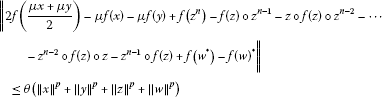(3.2)

for all $x,y,z,w\in A$ and all $\mu \in {\mathbb{T}}_{{n}_{0}}^{1}$. Then there exists a unique n-Jordan -derivation $D:A\to A$ such that

$\parallel f\left(x\right)-D\left(x\right)\parallel \le \frac{{2}^{p}\theta }{2-{2}^{p}}{\parallel x\parallel }^{p}$

for all $x\in A$.

Proof Letting $\phi \left(x,y,z,w\right)=\theta \left({\parallel x\parallel }^{p}+{\parallel y\parallel }^{p}+{\parallel z\parallel }^{p}+{\parallel w\parallel }^{p}\right)$ in Theorem 3.3, we have

$\parallel f\left(x\right)-D\left(x\right)\parallel \le \frac{{2}^{p}\theta }{2-{2}^{p}}{\parallel x\parallel }^{p}$

for all $x\in A$, as desired. □

Theorem 3.5 Let $f:A\to A$ be a mapping for which there exists a function $\phi :{A}^{4}\to \left[0,\mathrm{\infty }\right)$ satisfying (3.1) and (2.10). Then there exists a unique n-Jordan -derivation $D:A\to A$ satisfying (2.11).

Proof The proof is similar to the proofs of Theorems 2.4 and 3.3. □

Corollary 3.6 Let $f:A\to A$ be a mapping with $f\left(0\right)=0$ for which there exist positive constants θ and $p>n$ satisfying (3.2). Then there exists a unique n-Jordan -derivation $D:A\to A$ such that

$\parallel f\left(x\right)-D\left(x\right)\parallel \le \frac{{2}^{p}\theta }{{2}^{p}-2}{\parallel x\parallel }^{p}$

for all $x\in A$.

Proof Letting $\phi \left(x,y,z,w\right)=\theta \left({\parallel x\parallel }^{p}+{\parallel y\parallel }^{p}+{\parallel z\parallel }^{p}+{\parallel w\parallel }^{p}\right)$ in Theorem 3.5, we have

$\parallel f\left(x\right)-D\left(x\right)\parallel \le \frac{{2}^{p}\theta }{{2}^{p}-2}{\parallel x\parallel }^{p}$

for all $x\in A$, as desired. □

## References

1. Ulam SM: Problems in Modern Mathematics. Wiley, New York; 1940. Chapter VI

2. Hyers DH: On the stability of the linear functional equation. Proc. Natl. Acad. Sci. USA 1941, 27: 222–224.

3. Aoki T: On the stability of the linear transformation in Banach spaces. J. Math. Soc. Jpn. 1950, 2: 64–66.

4. Rassias TM: On the stability of the linear mapping in Banach spaces. Proc. Am. Math. Soc. 1978, 72: 297–300.

5. Cho Y, Saadati R, Vahidi J:Approximation of homomorphisms and derivations on non-Archimedean Lie ${C}^{\ast }$-algebras via fixed point method. Discrete Dyn. Nat. Soc. 2012., 2012: Article ID 373904

6. Cho Y, Eshaghi Gordji M, Zolfaghari S: Solutions and stability of generalized mixed type QC-functional equations in random normed spaces. J. Inequal. Appl. 2010., 2010: Article ID 403101

7. Eshaghi Gordji M, Bodaghi A, Alias IA: On the stability of quadratic double centralizers and quadratic multipliers: a fixed point approach. J. Inequal. Appl. 2011., 2011: Article ID 957541

8. Eshaghi Gordji M, Bodaghi A, Park C: A fixed point approach to the stability of double Jordan centralizers and Jordan multipliers on Banach algebras. Politehn. Univ. Bucharest Sci. Bull. Ser. A Appl. Math. Phys. 2011, 73(2):65–74.

9. Eshaghi Gordji M, Ghaemi MB, Kaboli Gharetapeh S, Shams S, Ebadian A:On the stability of ${J}^{\ast }$-derivations. J. Geom. Phys. 2010, 60: 454–459.

10. Eshaghi Gordji M, Khodaei H, Khodabakhsh R: General quartic-cubic-quadratic functional equation in non-Archimedean normed spaces. Sci. Bull. - “Politeh.” Univ. Buchar., Ser. a Appl. Math. Phys. 2010, 72(3):69–84.

11. Eshaghi Gordji M, Savadkouhi MB: Approximation of generalized homomorphisms in quasi-Banach algebras. An. Ştiinţ. Univ. “Ovidius” Constanţa Ser. Mat. 2009, 17(2):203–213.

12. Hyers DH, Isac G, Rassias TM Progress in Nonlinear Differential Equations and Their Applications. In Stability of Functional Equations in Several Variables. Birkhäuser Boston, Boston; 1998.

13. Hyers DH, Rassias TM: Approximate homomorphisms. Aequ. Math. 1992, 44: 125–153.

14. Isac G, Rassias TM: On the Hyers-Ulam stability of ψ -additive mapping. J. Approx. Theory 1993, 72: 131–137.

15. Isac G, Rassias TM: Stability of ψ -additive mapping. J. Math. Sci. 1996, 19: 219–228.

16. Jung S-M: Hyers-Ulam-Rassias Stability of Functional Equations in Mathematical Analysis. Hadronic Press, Palm Harbor; 2001.

17. Miura T, Takahasi SE, Hirasawa G: Hyers-Ulam-Rassias stability of Jordan homomorphisms on Banach algebras. J. Inequal. Appl. 2005, 2005: 435–441.

18. Park C: Hyers-Ulam-Rassias stability of homomorphisms in quasi-Banach algebras. Bull. Sci. Math. 2008, 132: 87–96.

19. Rassias TM, Semrl P: On the behavior of mapping which do not satisfy Hyers-Ulam stability. Proc. Am. Math. Soc. 1992, 114: 989–993.

20. Rassias TM: The problem of S. M. Ulam for approximately multiplicative mapping. J. Math. Anal. Appl. 2000, 246: 352–378.

21. Eshaghi Gordji, M: Nearly n-Jordan derivations. Preprint

22. Ebadian, A: Approximately n-Jordan homomorphisms: a fixed point approach. Preprint

23. Eshaghi Gordji, M: On approximate n-ring homomorphisms and n-ring derivations. Nonlinear Funct. Anal. Appl. (to appear)

24. Eshaghi Gordji M, Ghobadipour N, Park C:Jordan -homomorphisms on ${C}^{\ast }$-algebras. Oper. Matrices 2011, 5: 541–551.

25. Hejazian S, Mirzavaziri M, Moslehian MS: n -homomorphisms. Bull. Iran. Math. Soc. 2005, 31: 13–23.

26. Park C:Homomorphisms between Poisson $J{C}^{\ast }$-algebras. Bull. Braz. Math. Soc. 2005, 36: 79–97.

27. Eshaghi Gordji M, Fazeli A:Stability and superstability of homomorphisms on ${C}^{\ast }$-ternary algebras. An. Ştiinţ. Univ. “Ovidius” Constanţa Ser. Mat. 2012, 20: 173–188.

## Author information

Authors

### Corresponding author

Correspondence to Choonkil Park.

### Competing interests

The authors declare that they have no competing interests.

### Authors’ contributions

All authors conceived of the study, participated in its design and coordination, drafted the manuscript, participated in the sequence alignment, and read and approved the final manuscript.

## Rights and permissions

Reprints and Permissions

Park, C., Ghaffary Ghaleh, S. & Ghasemi, K. Approximate n-Jordan -derivations on ${C}^{\ast }$-algebras and $J{C}^{\ast }$-algebras. J Inequal Appl 2012, 273 (2012). https://doi.org/10.1186/1029-242X-2012-273

• Accepted:

• Published:

• DOI: https://doi.org/10.1186/1029-242X-2012-273

### Keywords

• n-Jordan -derivation
• ${C}^{\ast }$-algebra
• $J{C}^{\ast }$-algebra
• Hyers-Ulam stability